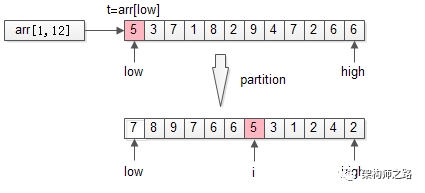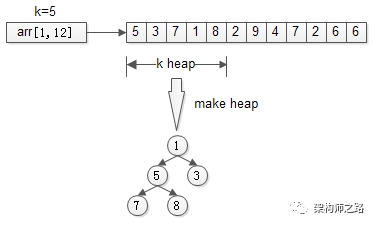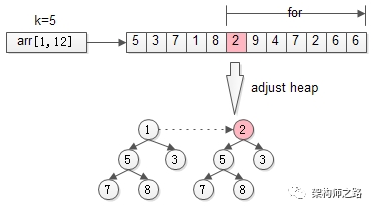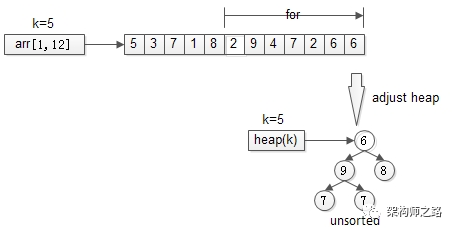partition使用第一个元素t=arr[low]为哨兵，把数组分成了两个半区：

• 左半区比t大

• 右半区比t小

1. 外层for循环

2. 内层for循环

3. 最内层的swap

O(n) O(n) O(1) = O(n^2)1. 新建堆

2. for循环

3. 调整堆

O(k) + O(n) O(lg(k)) = O(nlg(k))

• 当n=1时，sum函数只计算1次

f(1)=1【式子A】

• f(n)的计算次数，等于f(n-1)的计算次数，再加1次计算

f(n)=f(n-1)+1【式子B】

【式子B】不断的展开，再配合【式子A】：

f(n)=f(n-1)+1

f(n-1)=f(n-2)+1

f(2)=f(1)+1

f(1)=1

f(n)+f(n-1)+…+f(2)+f(1)

=

[f(n-1)+1]+[f(n-2)+1]+…+[f(1)+1]+

f(n)=n

• 当n=1时，bs函数只计算1次

f(1)=1【式子A】

• f(n)的计算次数，等于f(n/2)的计算次数，再加1次计算

f(n)=f(n/2)+1【式子B】

【式子B】不断的展开，

f(n)=f(n/2)+1

f(n/2)=f(n/4)+1

f(n/4)=f(n/8)+1

f(n/2^(m-1))=f(n/2^m)+1

f(n)+f(n/2)+…+f(n/2^(m-1))
=
[f(n/2)+1]+[f(n/4)+1]+…+[f(n/2^m)]+

f(n)=f(n/2^m)+m

f(1)=1

f(n)=1+lg(n)

• 当n=1时，quick_sort函数只计算1次

f(1)=1【式子A】

f(n)=n+f(n/2)+f(n/2)=n+2*f(n/2)【式子B】

(1)partition本质是一个for，计算次数是n；

(2)二分查找只需要递归一个半区，而快速排序左半区和右半区都要递归，这一点在分治法减治法一章节已经详细讲述过；

【式子B】不断的展开，

f(n)=n+2*f(n/2)

f(n/2)=n/2+2*f(n/4)

f(n/4)=n/4+2*f(n/8)

f(n/2^(m-1))=n/2^(m-1)+2f(n/2^m)

f(n)=n+2*f(n/2)

=n+2[n/2+2\f(n/4)]=2n+4*f(n/4)

=2n+4[n/4+2f(n/8)]=3n+8f(n/8)

=…

=m*n+2^m*f(n/2^m)

f(1)=1

f(n)=lg(n)*n+2^(lg(n))*f(1)=n*lg(n)+n

wacalei，有点意思哈！

• for循环的时间复杂度往往是O(n)

• 树的高度的时间复杂度往往是O(lg(n))

• 二分查找的时间复杂度是O(lg(n))，快速排序的时间复杂度n*(lg(n))

• 递归求解，未来再问时间复杂度，通杀

0%